# imdct

Inverse modified discrete cosine transform

## Syntax

``X = imdct(Y,win)``
``X = imdct(Y,win,Name,Value)``

## Description

example

````X = imdct(Y,win)` returns the inverse modified discrete cosine transform (IMDCT) of `Y`, followed by multiplication with time window `win` and overlap-addition of the frames with 50% overlap.```
````X = imdct(Y,win,Name,Value)` sets each property `Name` to the specified `Value`. Unspecified properties have default values.```

## Examples

collapse all

Read in an audio file, convert it to mono, and then plot it.

```audioIn = audioread('FunkyDrums-44p1-stereo-25secs.mp3'); audioIn = mean(audioIn,2); figure(1) plot(audioIn,'bo') ylabel('Amplitude') xlabel('Sample Number')```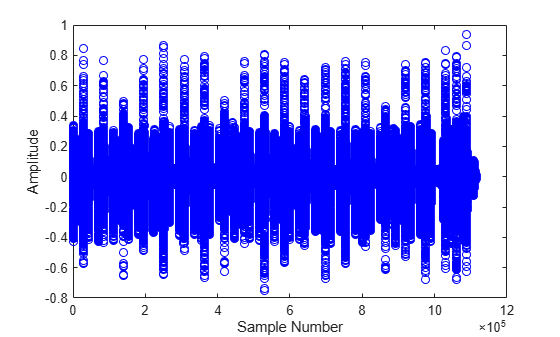Calculate the MDCT using a 4096-point sine window. Plot the power of the MDCT coefficients over time.

```N = 4096; wdw = sin(pi*((1:N)-0.5)/N); C = mdct(audioIn,wdw); figure(2) surf(pow2db(C.*conj(C)),'EdgeColor','none'); view([0 90]) xlabel('Frame') ylabel('Frequency') axis([0 size(C,2) 0 size(C,1)]) colorbar```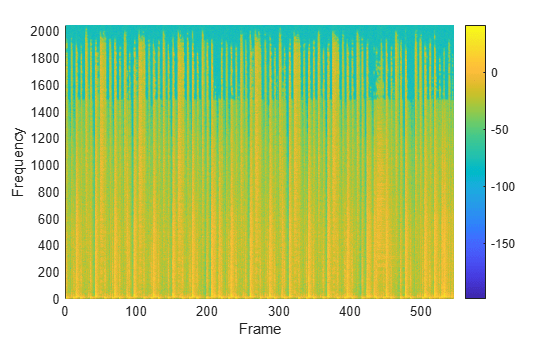Transform the representation back to the time domain. Verify the perfect reconstruction property by computing the mean squared error. Plot the reconstructed signal over the original signal.

```audioReconstructed = imdct(C,wdw); err = mean((audioIn-audioReconstructed(1:size(audioIn,1),:)).^2)```
```err = 9.5937e-31 ```
```figure(1) hold on plot(audioReconstructed,'r.') ylabel('Amplitude') xlabel('Sample Number')```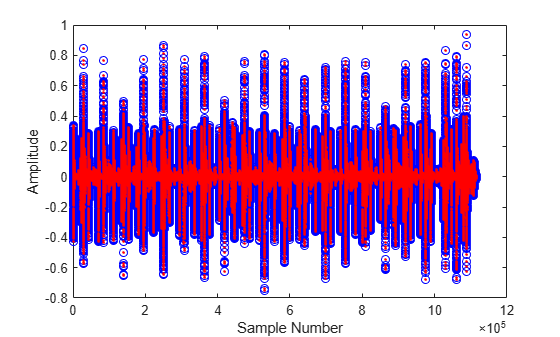To enable perfect reconstruction, the `mdct` function zero-pads the front and back of the audio input signal. The signal returned from `imdct` removes the zero padding added for perfect reconstruction.

Read in an audio file, create a 2048-point Kaiser-Bessel-derived window, and then clip the audio signal so that its length is a multiple of 2048.

```[x,fs] = audioread('Click-16-44p1-mono-0.2secs.wav'); win = kbdwin(2048); xClipped = x(1:end - rem(size(x,1),numel(win))); ```

Convert the signal to the frequency domain, and then reconstruct it back in the time domain. Plot the original and reconstructed signals and display the reconstruction error.

```C = mdct(xClipped,win); y = imdct(C,win); figure(1) t = (0:size(xClipped,1)-1)'/fs; plot(t,xClipped,'bo',t,y,'r.') legend('Original Signal','Reconstructed Signal') title(strcat("Reconstruction Error = ",num2str(mean((xClipped-y).^2)))) xlabel('Time (s)') ylabel('Amplitude') ```You can perform the MDCT and IMDCT without input padding using the `PadInput` name-value pair. However, there will be a reconstruction error in the first half-frame and last half-frame of the signal.

```C = mdct(xClipped,win,'PadInput',false); y = imdct(C,win,'PadInput',false); figure(2) t = (0:size(xClipped,1)-1)'/fs; plot(t,xClipped,'bo',t,y,'r.') legend('Original Signal','Reconstructed Signal') title(strcat("Reconstruction Error (Without Input Padding) = ",num2str(mean((xClipped-y).^2)))) xlabel('Time (s)') ylabel('Amplitude') ```If you specify an input signal to the `mdct` that is not a multiple of the window length, then the input signal is padded with zeros. Pass the original unclipped signal through the transform pair and compare the original signal and the reconstructed signal.

```C = mdct(x,win); y = imdct(C,win); figure(3) subplot(2,1,1) plot(x) title('Original Signal') ylabel('Amplitude') axis([0,max(size(y,1),size(x,1)),-0.5,0.5]) subplot(2,1,2) plot(y) title('Reconstructed Signal') xlabel('Time (s)') ylabel('Amplitude') axis([0,max(size(y,1),size(x,1)),-0.5,0.5]) ```The reconstructed signal is padded with zeros at the back end. Remove the zero-padding from the reconstructed signal, plot the original and reconstructed signal, and then display the reconstruction error.

```figure(4) y = y(1:size(x,1)); t = (0:size(x,1)-1)'/fs; plot(t,x,'bo',t,y,'r.') legend('Original Signal','Reconstructed Signal') title(strcat("Reconstruction Error = ",num2str(mean((x-y).^2)))) xlabel('Time (s)') ylabel('Amplitude') ```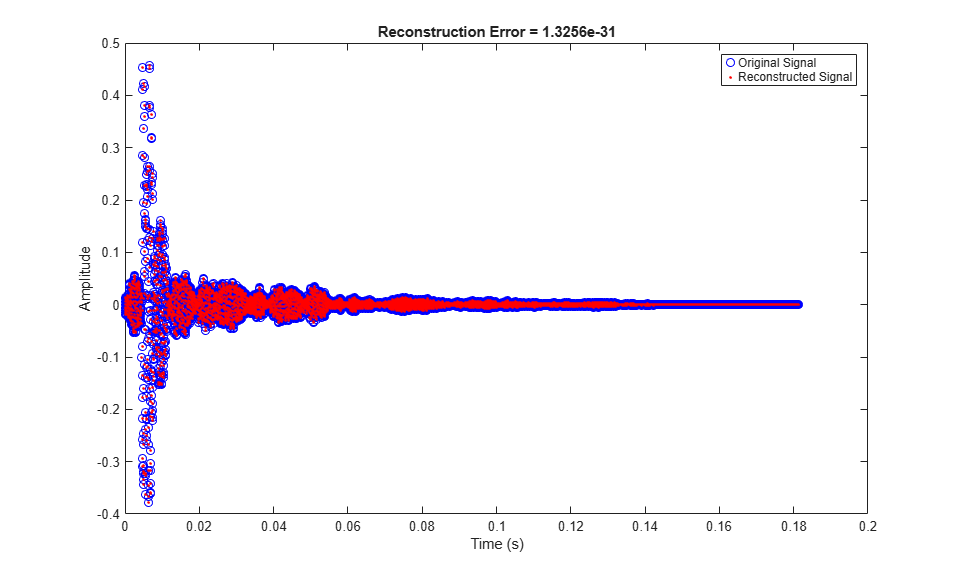Create a `dsp.AudioFileReader` object to read in audio data frame-by-frame. Create a `dsp.SignalSink` to log the reconstructed signal for comparison. Create a `dsp.AsyncBuffer` to buffer the input stream.

```fileReader = dsp.AudioFileReader('FunkyDrums-44p1-stereo-25secs.mp3'); logger = dsp.SignalSink; buff = dsp.AsyncBuffer;```

Create a 512-point Kaiser-Bessel-derived window.

```N = 512; win = kbdwin(N);```

In an audio stream loop:

1. Read a frame of data from the file.

2. Write the frame of data to the async buffer.

3. If half a frame of data is present, read from the buffer and then perform the transform pair. Overlap-add the current output from `imdct` with the previous output, and log the results. Update the memory.

```mem = zeros(N/2,2); % initialize an empty memory while ~isDone(fileReader) audioIn = fileReader(); write(buff,audioIn); while buff.NumUnreadSamples >= N/2 x = read(buff,N,N/2); C = mdct(x,win,'PadInput',false); y = imdct(C,win,'PadInput',false); logger(y(1:N/2,:)+mem) mem = y(N/2+1:end,:); end end % Perform the transform pair one last time with a zero-padded final signal. x = read(buff,N,N/2); C = mdct(x,win,'PadInput',false); y = imdct(C,win,'PadInput',false); logger(y(1:N/2,:)+mem) reconstructedSignal = logger.Buffer;```

Read in the entire original audio signal. Trim the front and back zero padding from the reconstructed signal for comparison. Plot one channel of the original and reconstructed signals and display the reconstruction error.

```[originalSignal,fs] = audioread(fileReader.Filename); signalLength = size(originalSignal,1); reconstructedSignal = reconstructedSignal((N/2+1):(N/2+1)+signalLength-1,:); t = (0:size(originalSignal,1)-1)'/fs; plot(t,originalSignal(:,1),'bo',t,reconstructedSignal(:,1),'r.') legend('Original Signal','Reconstructed Signal') title(strcat("Reconstruction Error = ", ... num2str(mean((originalSignal-reconstructedSignal).^2,'all')))) xlabel('Time (s)') ylabel('Amplitude')```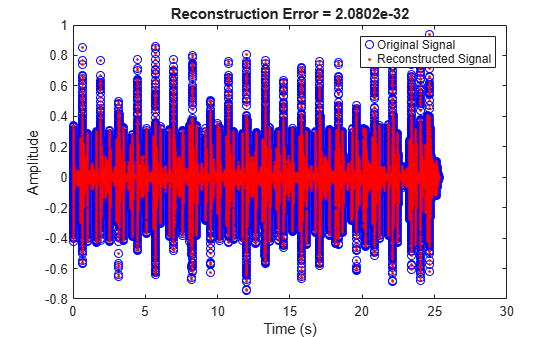## Input Arguments

collapse all

Modified discrete cosine transform (MDCT), specified as a vector, matrix, or 3-D array. The dimensions of `Y` are interpreted as output from the `mdct` function. If `Y` is an L-by-M-by-N array, the dimensions are interpreted as:

• L –– Number of points in the frequency-domain representation of each frame. L must be half the number of points in the window, `win`.

• M –– Number of frames.

• N –– Number of channels.

Data Types: `single` | `double`

Window applied in the time domain, specified as vector. The length of win must be twice the number of rows of `Y`: `numel(win)==2*size(Y,1)`. To enable perfect reconstruction, use the same window used in the forward transformation `mdct`.

Data Types: `single` | `double`

### Name-Value Pair Arguments

Specify optional comma-separated pairs of `Name,Value` arguments. `Name` is the argument name and `Value` is the corresponding value. `Name` must appear inside quotes. You can specify several name and value pair arguments in any order as `Name1,Value1,...,NameN,ValueN`.

Example: `'PadInput',false`

Flag if input to the forward `mdct` was padded. If set to `true`, the output is truncated at both ends to remove the zero-padding that the forward `mdct` added.

Data Types: `logical`

## Output Arguments

collapse all

Inverse modified discrete cosine transform (IMDCT) of input array `Y`, returned as a column vector or matrix of independent channels.

Data Types: `single` | `double`

## Algorithms

The inverse modified discrete cosine transform is a time-frequency transform. Given a frequency domain input signal `Y` and window `win`, the `imdct` function performs the follows steps for each independent channel:

1. Each frame of the input is converted into a time-domain representation:

`$X\left(n\right)=\sum _{k=0}^{\frac{N}{2}-1}Y\left(k\right)\mathrm{cos}\left[\frac{\pi }{\left(N}{2}\right)}\left(n+\frac{\left(N}{2}\right)+1}{2}\right)\left(k+\frac{1}{2}\right)\right]\text{\hspace{0.17em}},\text{ }n=0,1,...,N-1$`

where N is the number of elements in `win`.

2. Each frame of the time-domain signal is multiplied by the window, `win`.

3. The frames are overlap-added with 50% overlap to construct a contiguous time-domain signal. If `PadInput` is set to true, the `imdct` function assumes the original input signal in the forward transform (`mdct`) was padded with N/2 zeros on the front and back and removes the padding. By default, `PadInput` is set to `true`.

 Princen, J., A. Johnson, and A. Bradley. "Subband/Transform Coding Using Filter Bank Designs Based on Time Domain Aliasing Cancellation." IEEE International Conference on Acoustics, Speech, and Signal Processing (ICASSP). 1987, pp. 2161–2164.

 Princen, J., and A. Bradley. "Analysis/Synthesis Filter Bank Design Based on Time Domain Aliasing Cancellation." IEEE Transactions on Acoustics, Speech, and Signal Processing. Vol. 34, Issue 5, 1986, pp. 1153–1161.

## SupportGet trial now